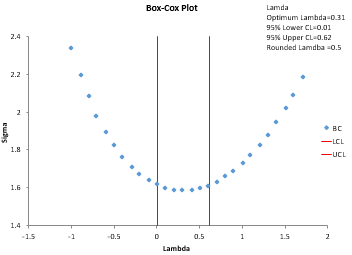•You need to do a process capability analysis, but your data are not normally distributed

• You need to run an experiment to determine if the means of two processes are the same - but the data are not normally distributed and the statistical test requires a normal distribution

What do you do?  You transform the data using either the Box-Cox transformation or the Johnson transformation.

## About the Box-Cox and Johnson Transformations and SPC for Excel

Having data that are normally distributed often simplifies your life.  Many statistical tests are based on the assumption that your data are normally distributed.  The calculation of Cpk (process capability) values require a normal distribution.  If you data are not normally distributed, the first thing to try is to transform the data into a normal distribution using either the Box-Cox transformation or the Johnson transformation.

The Box-Cox transformation is Yλ where λ is value between -5 and 5.  The procedure is designed to find the value of λ that minimizes the variation (standard deviation).  For example, if λ = 2 minimizes the variation, then the data would be transformed as Y2.  The Johnson transformation is more complex than the Box-Cox transformation.  The Johnson transformation is chosen from three different functions by changing four parameters.

## Box-Cox Transformation Features

• Individual or subgroup values
• Option to use standard deviation estimated  from pooled variance, subgroup average range, or subgroup average standard deviation
• Optimum lambda or round lambda
• Descriptive statistics of the original and transformed data including Anderson-Darling statistic and associated p-value
• Histograms of original data and transformed data
• P-P plot for original data and transformed data
• Print out of transformed data values

## Johnson Transformation Features

• Plot the z value versus p-value for each of the three Johnson distributions (SB, SU, SL)
• Descriptive statistics of the original and transformed data including Anderson-Darling statistic and associated p-value
• Histograms of original data and transformed data
• P-P plot for original data and transformed data
• Print out of transformed data values

#### SPC Knowledge BaseSign up for our FREE monthly publication featuring SPC techniques and other statistical topics.